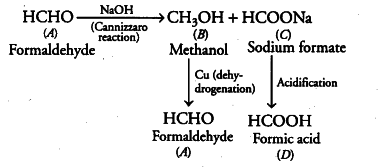# Two moles of an organic compound A on treatment with a strong base gives two compounds B and C

Two moles of an organic compound A on treatment with a strong base gives two compounds B and C. Compound B on dehydrogenation with Cu gives A while acidification of C yields carboxylic acid D with molecular formula . Identify the compounds A, B, C and D and write all the chemical reactions involved.

Since, the molecular formula of D thus, D is HCOOH (formic acid). D is obtained by the acidification of
C, so, C is sodium formate (HCOONa).
Thus, A must be formaldehyde (as it undergoes Cannizzaro reaction with a strong base).Thus, A = Formaldehyde (HCHO)
B = Methanol
C = Sodium formate (HCOONa) D = Formic acid (HCOOH)